# Causal Learner: A Toolbox for Causal Structure and Markov Blanket Learning

Written by Zhaolong Ling and Kui Yu.

## Overview

Causal Learner is a toolbox for learning causal structure and Markov blanket (MB) from data. It integrates functions for generating simulated Bayesian network (BN) data, a set of state-of-the-art global causal structure learning algorithms, a set of state-of-the-art local causal structure learning algorithms, a set of state-of-the-art MB learning algorithms, and functions for evaluating algorithms. Figure 1 gives examples of a global causal structure, local causal structure, and MB (T in black is a target node). The data generation part of Causal Learner is written in R, and the rest of Causal Learner is written in MATLAB. Causal Learner aims to provide researchers and practitioners with an open-source platform for causal learning from data and for the development and evaluation of new causal learning algorithms.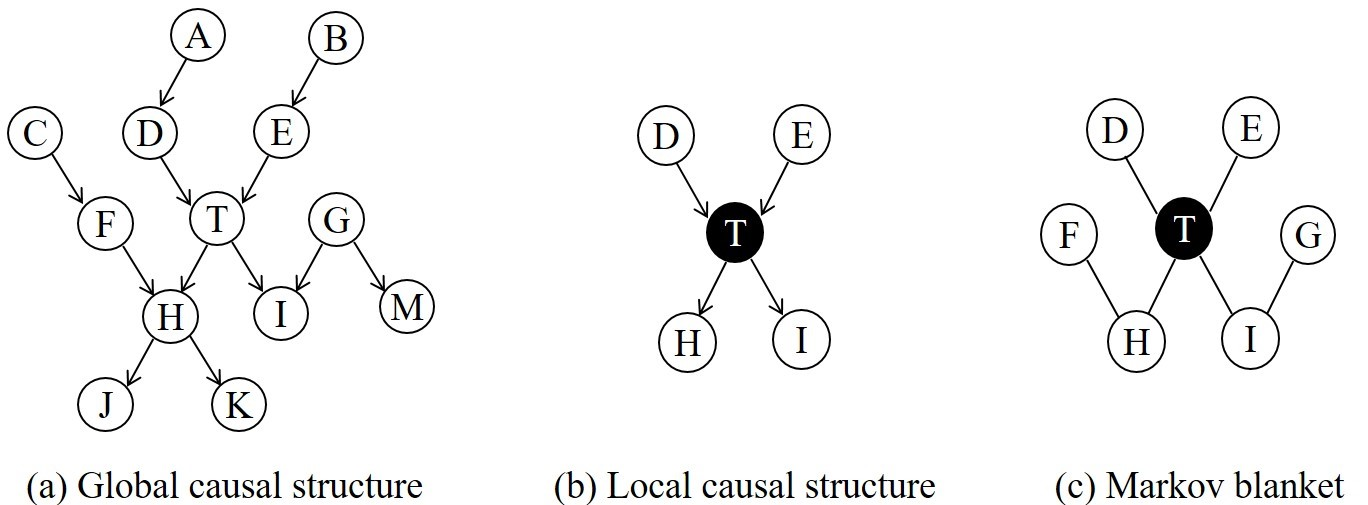Figure 1: Examples of a global causal structure, local causal structure, and Markov blanket.

## Architecture

Figure 2 shows the hierarchical architecture of Causal Learner, in comparison with Causal Explorer. As Causal Explorer represents the state-of-the-art ten years ago, it does not contain many new algorithms, and it does not have a data generation or evaluation component. By contrast, Causal Learner conceives a more ambitious blueprint. It aims to support the entire causal structure and MB learning procedure, including data generation, state-of-the-art algorithms, and algorithm evaluation.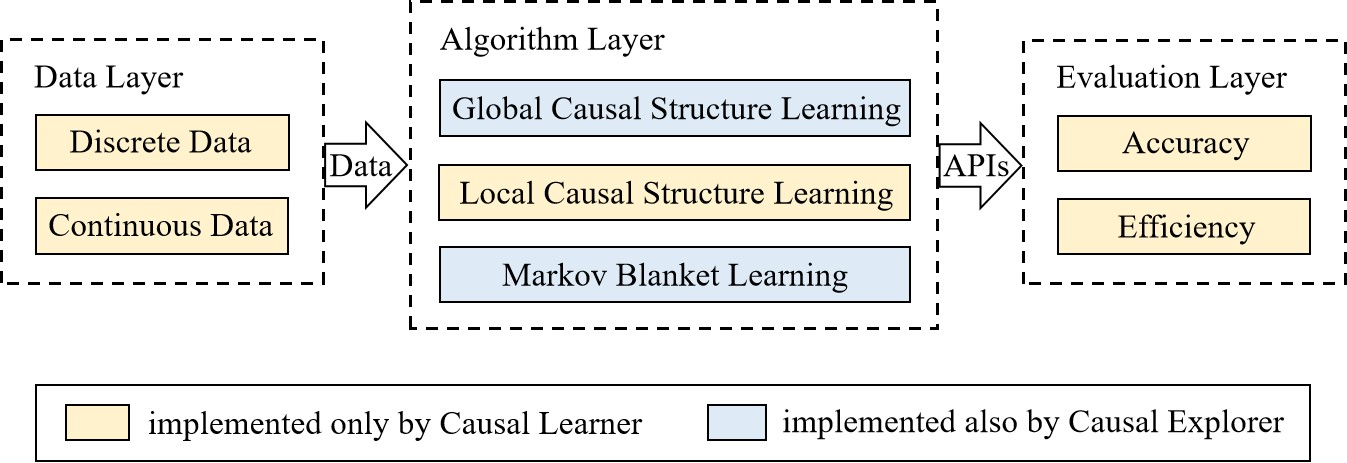Figure 2: The architecture of Causal Learner.

## Data

In the data layer, Causal Learner generates two types of data: discrete data and continuous data. The data are generated based on various benchmark BNs (written in R), and the details of each BN are shown in Table 1. The data can also be generated by the bnlearn toolbox, but the generated data are encapsulated in R language classes and cannot be easily used by researchers using other programming languages. Causal Learner can output the generated data as text for easy and flexible use.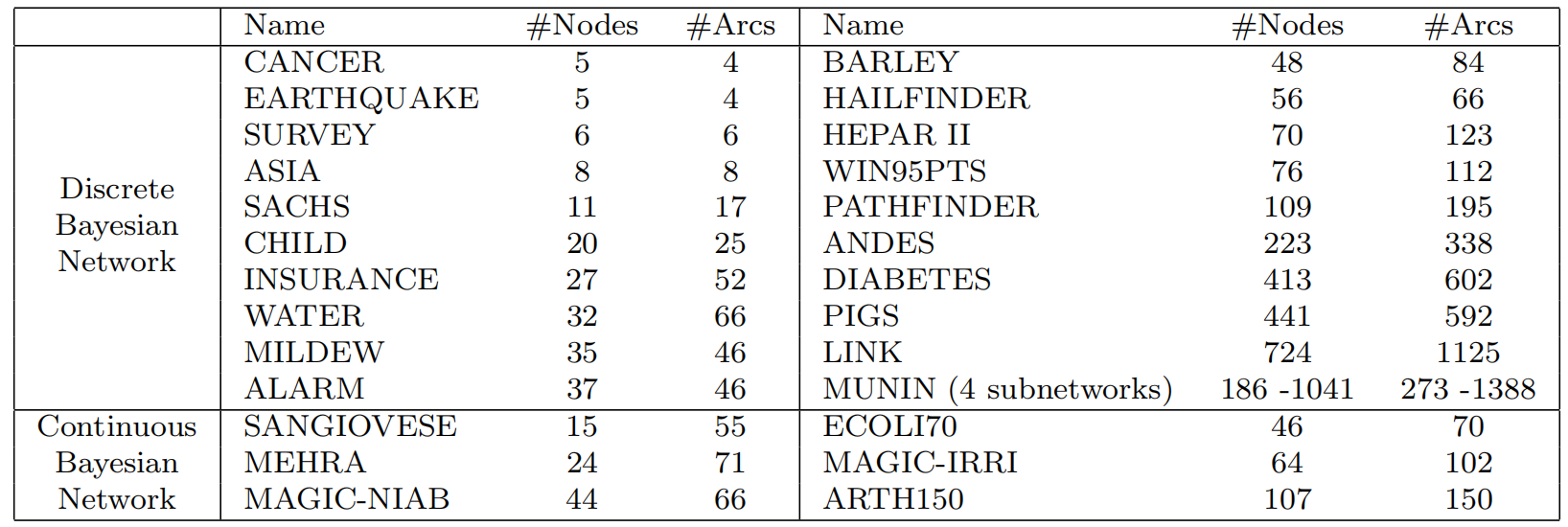Table 1: Benchmark Bayesian networks.

## Algorithm

In the algorithm layer, Causal Learner implements 7 global causal structure learning algorithms, 4 local causal structure learning algorithms, and 15 MB learning algorithms (written in MATLAB). Table 2 lists all of these algorithms. To ensure the correctness of the algorithms implemented in Causal Learner, unless the original implementations of the algorithms are not released, we always try to integrate the original versions rather than re-implement them. Additionally, we have used the same data to evaluate the algorithms in Causal Learner. Compared with Causal Explorer, the results of Causal Learner are comparable in accuracy and much more efficient.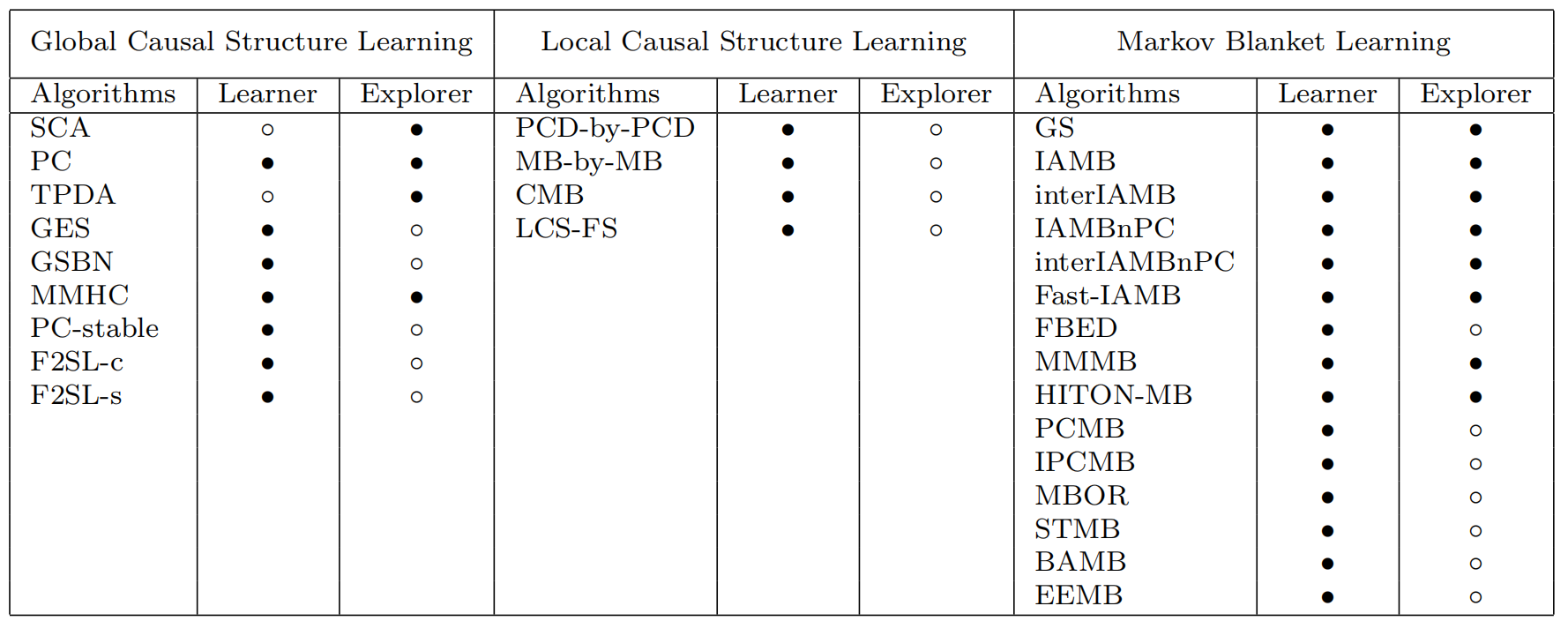Table 2: Algorithms included in (indicated by •) and absent from (indicated by ◦) Causal Learner and Causal Explorer.

## Evaluation

In the evaluation layer, Causal Learner provides abundant metrics for evaluating causal structure and MB learning algorithms (written in MATLAB). In terms of accuracy, global and local causal structure learning algorithms are evaluated using the same 7 metrics: Ar_F1 (Ar denotes arrow), Ar_precision, Ar_recall, SHD, Miss, Extra, and Reverse. MB learning algorithms are evaluated using 3 metrics: Adj_F1 (Adj denotes adjacent), Adj_precision, and Adj_recall. In terms of efficiency, global causal structure learning algorithms are evaluated using running time, while both local causal structure and MB learning algorithms are evaluated using running time and the number of conditional independence tests.

## Usage Example

Causal Learner comes with a manual that details the BNs, algorithms, evaluation metrics, and how each function is used. Figure 3 shows an example of global causal structure learning using Causal Learner. As shown in the figure, Causal Learner needs only 4 input parameters when learning a global causal structure, while Causal Explorer requires additional parameters such as “domain count”. Thus, Causal Learner uses a cleaner input format than that of Causal Explorer.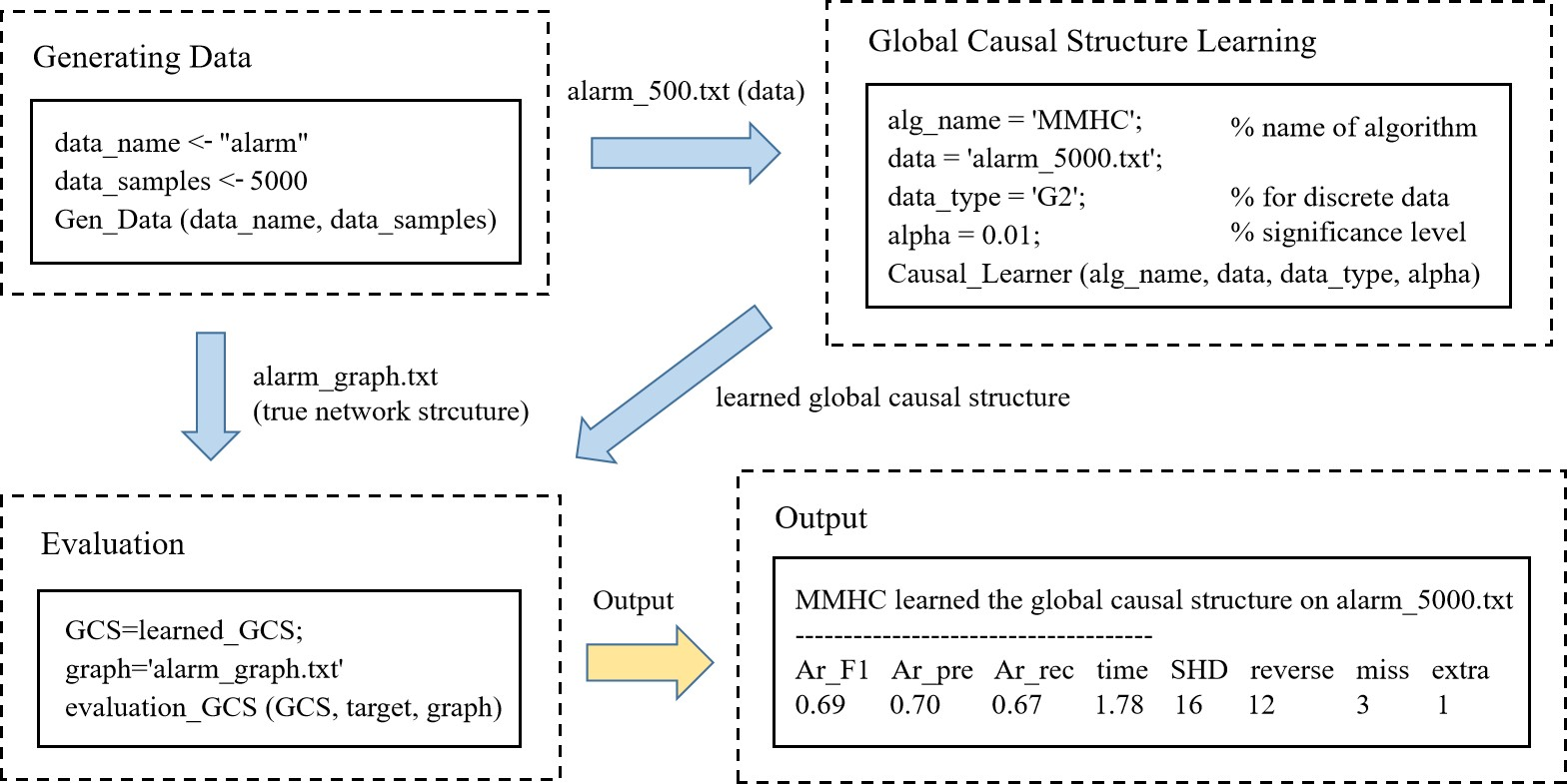Figure 3: An example of using Causal Learner to learn a global causal structure.